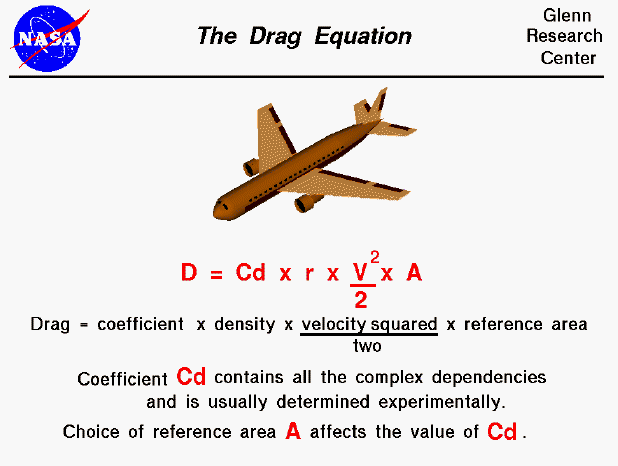Drag depends on the density of the air, the square of the velocity, the air's viscosity and compressibility, the size and shape of the body, and the body's inclination to the flow. In general, the dependence on body shape, inclination, air viscosity, and compressibility is very complex.

One way to deal with complex dependencies is to characterize the dependence by a single variable. For drag, this variable is called the drag coefficient, designated "Cd." This allows us to collect all the effects, simple and complex, into a single equation. The drag equation states that drag D is equal to the drag coefficient Cd times the density r times half of the velocity V squared times the reference area A.

D = Cd * A * .5 * r * V^2

For given air conditions, shape, and inclination of the object, we must determine a value for Cd to determine drag. Determining the value of the drag coefficient is more difficult than determining the lift coefficient because of the multiple sources of drag. The drag coefficient given above includes form drag, skin friction drag, wave drag, and induced drag components. (Ram drag is usually included in the net thrust because it depends on the airflow through the engine.) Drag coefficients are almost always determined experimentally using a wind tunnel.

Notice that the area (A) given in the drag equation is given as a reference area. The drag depends directly on the size of the body. Since we are dealing with aerodynamic forces, the dependence can be characterized by some area. But which area do we choose? If we think of drag as being caused by friction between the air and the body, a logical choice would be the total surface area of the body. If we think of drag as being a resistance to the flow, a more logical choice would be the frontal area of the body that is perpendicular to the flow direction. And finally, if we want to compare with the lift coefficient, we should use the same wing area used to derive the lift coefficient. Since the drag coefficient is usually determined experimentally by measuring drag and the area and then performing the division to produce the coefficient, we are free to use any area that can be easily measured. If we choose the wing area, rather than the cross-sectional area, the computed coefficient will have a different value. But the drag is the same, and the coefficients are related by the ratio of the areas. In practice, drag coefficients are reported based on a wide variety of object areas. In the report, the aerodynamicist must specify the area used; when using the data, the reader may have to convert the drag coefficient using the ratio of the areas.

In the equation given above, the density is designated by the letter "r." We do not use "d" for density since "d" is often used to specify distance. In many textbooks on aerodynamics, density is given by the Greek symbol "rho" (Greek for "r"). The combination of terms "density times the square of the velocity divided by two" is called the dynamic pressure and appears in Bernoulli's pressure equation.Guided Tours

Beginner's Guide to Aerodynamics
Beginner's Guide to Propulsion
Beginner's Guide to Model Rockets
Beginner's Guide to Kites
Beginner's Guide to Aeronautics